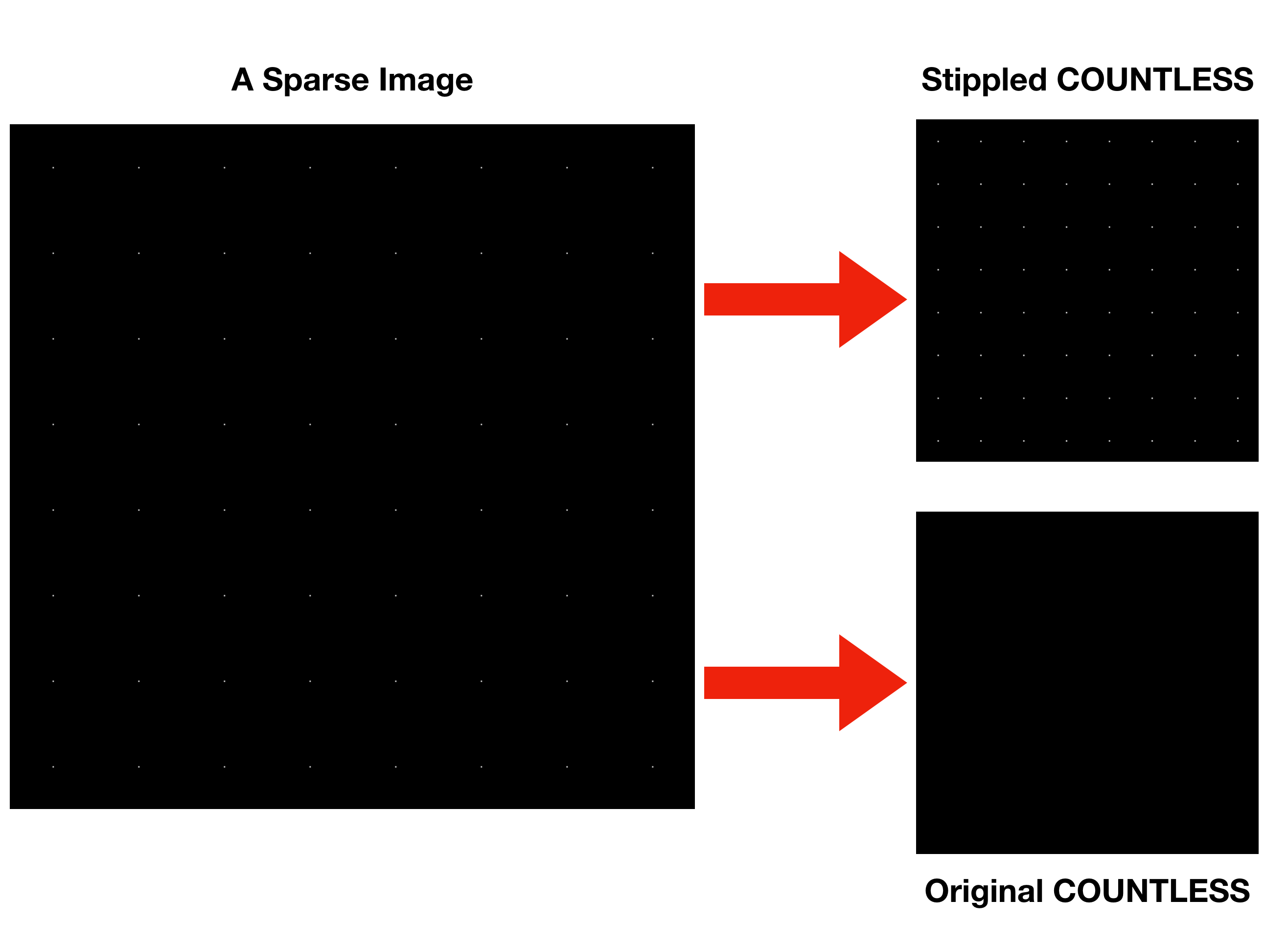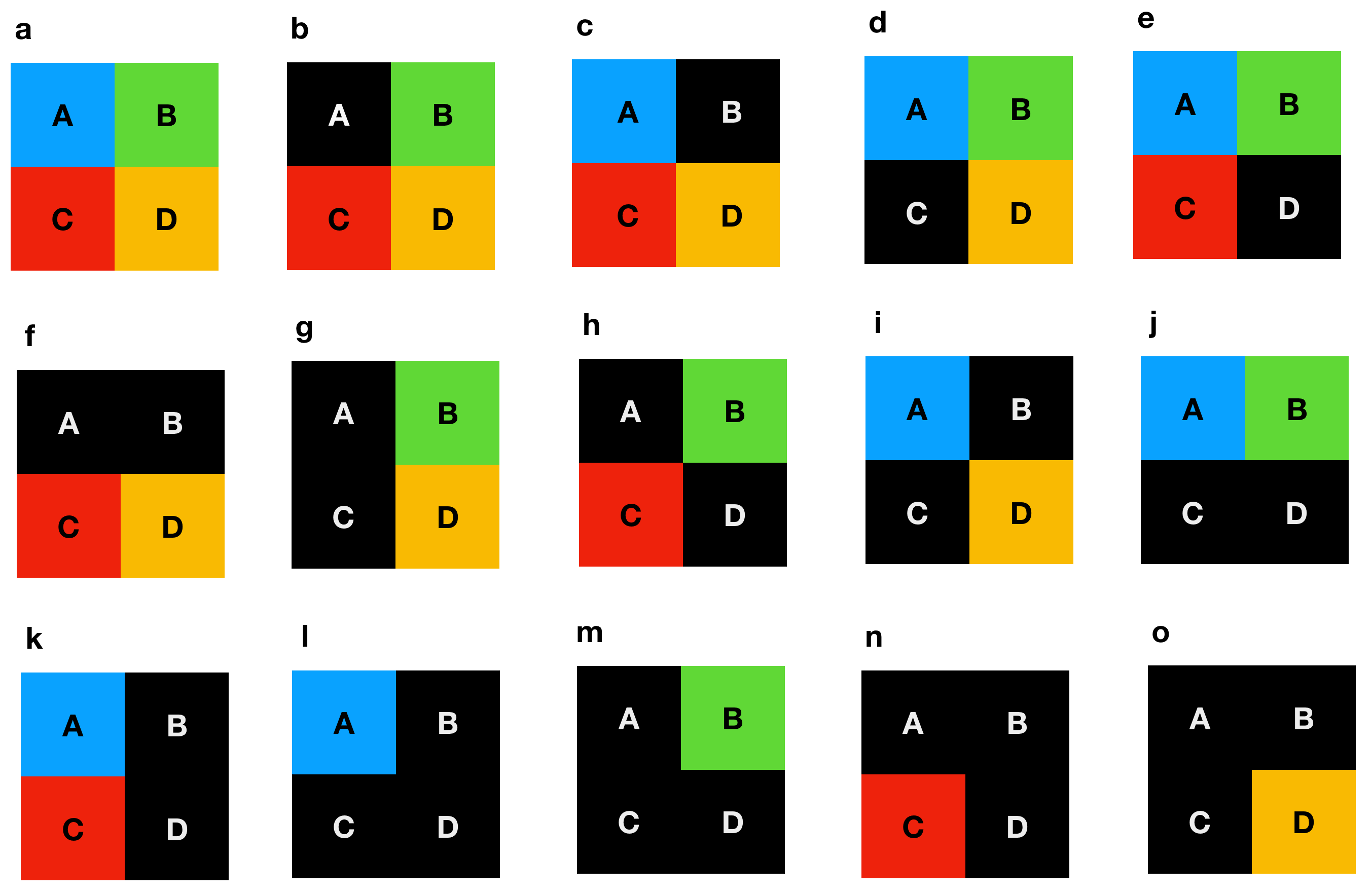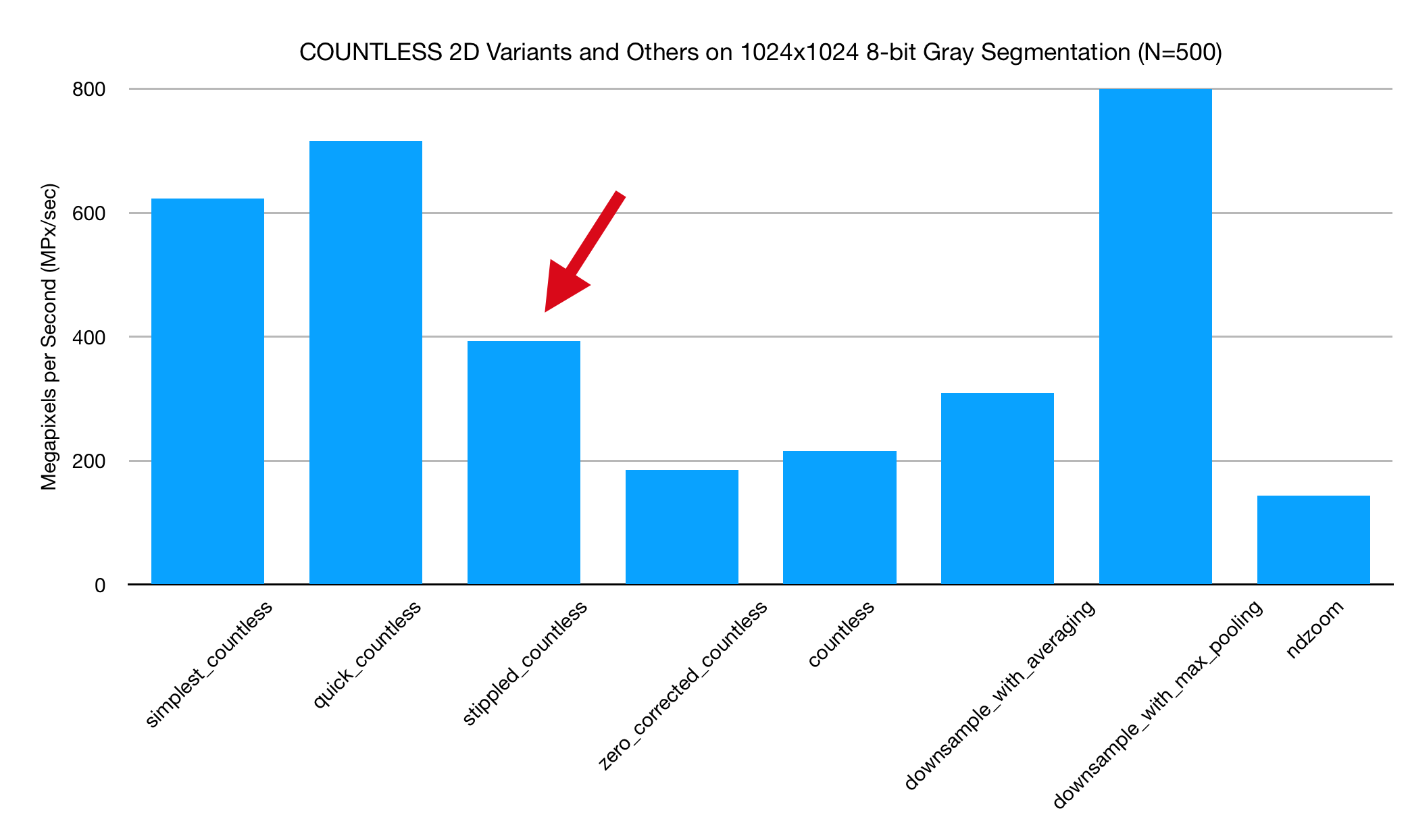# Stippled COUNTLESS 2D — 2x Downsampling of Sparsely Labeled ImagesOriginal COUNTLESS erodes sparse data on a black field. Note that the field labeled “Original COUNTLESS” is pure black while the faint points are retained in the Stippled COUNTLESS field.

From time to time a scientific Python user will tell me that they’d like to use COUNTLESS 2D, but they’re working with a sparse dataset. For instance, they might have a volume of cells and they’d like to paint a dot over each cell body’s center of mass. However, if they were to use COUNTLESS 2D, the surrounding numerous black pixels would erase the small dots within a small number of downsamples, leaving the canvas entirely black when zoomed out.

If the dot size is a single pixel and will never be adjacent to another pixel (even when downsampled), then a simple solution is to use `np.maximum`. However, if there is a chance that your labels might collide, it makes more sense to treat the surrounding black pixels as background and proceed with choosing the mode of each 2x2 block.

# Algorithm

Stippled COUNTLESS can be stated simply. Let the four pixels of a 2x2 block be labeled A, B, C, and D.

1. If there’s a non-zero pair match among A,B, and C return that value.
2. If there is no non-zero pair match between A, B, or C pick D unless D is zero.
3. If D is zero, try A, then B, then C until a non-zero value is found or all elements have been tried in which case return zero.

The discussion below describes why this is correct.Figure 1. A non-exhaustive enumeration of different situations that may be encountered now that zero (black) is treated as a separate category. (a) all values are different (b-e) A single black pixel rotates clockwise around the grid (f-k) covering of two black pixels (l-o) coverings of three black pixels. Other situations not shown: all black, matches between non-zero values.

Recall the most important lines of the COUNTLESS 2D algorithm:

`# LISTING 1: COUNTLESS 2D Algorithm1. def countless2d(a, b, c, d):  2.    ab_ac = a * ((a == b) | (a == c))  3.    bc = b * (b == c) 4.    abc = ab_ac | bc 5.    return abc + (abc == 0) * d`

First consider situation (b) from Figure 1 where only A is black. On Line 2, if A is zero and B,C are nonzero, then `ab_ac` is zero, Line 3 will pick B if B and C match else it will also be zero, in which case D will be chosen on Line 5.

Second, consider situation (j) where C and D are black. If A does not match B, it can’t match C, and thus `ab_ac` is zero. B does not match C, so Line 3 is zero. D will be chosen, but D is also zero when we really wanted either A or B to be picked. What a dilemma.

Case (n), where A, B, and D are zero, is also nefarious. A matches B but evaluates to zero, B doesn’t match C, and D is chosen, but we really wanted C!

Fortunately, there is a simple solution. I’ll derive it by treating increasingly complex cases from single black pixels, then double black, then to triple and quadruple black.

In the case of the single black pixel, the original algorithm does the right thing in cases (b), (c), and (d). It either picks D if there are no matches, or AB, AC, or BC if there is a match. At first glance it appears that (b) might pose a problem because factor A is zero, but luckily Line 2 would produce zero anyway because it differs from B and C.

Case (e) provides the first real challenge. If there are no matches among A, B, and C, D will be chosen, but we’d like to have picked either A, B, or C instead. This can be remedied by inserting A if D is zero.

`# LISTING 2: Single Black Pixel Solution# Modified Line 55.     return abc + (abc == 0) * (d + (d == 0) * a)`

The double black pixels pose more of a challenge but they also provide some relief at the same time. It’s now possible for A to be zero while matching B, C, or D, for B to be zero while matching C, and for D to be zero at the same time as another pixel. However, if two pixels are black, we can choose any of the non-zero pixels as they will either be different (making the choice arbitrary) or matching (making the choice identical).

Our original algorithm correctly handles cases (f), (g), and (i). Our modified algorithm correctly handles cases (f), (g), (i), (j), and (k). However, it misses case (h). This is because (h) is zeroed at both A and D. If B matches C, it will be handled correctly, but if B and C don’t match the algorithm will return zero when it should return one of them.

We can modify our algorithm some more. Instead of only checking D then A, why not check D, A, and B?

`# LISTING 3: Double Black Pixel Solution# Insert an extra line before the return5.    nonzero = a + (a == 0) * b6.    return abc + (abc == 0) * (d + (d == 0) * nonzero)`

This solves case (h) for us by selecting B in the case of a non-match. It doesn’t interfere with the other examples because this logic only has an impact if zero is about to be returned.

The triple black makes things even tougher. Consider case (n), which is case (h) with B blacked out. However, it looks like our modified algorithm already handles cases (l), (m), and (o) already! The original algorithm would have only handled case (o) properly. Let’s add in logic for case (n).

`# LISTING 4: Triple Black Pixel Solution# Insert an extra line before the return5.    nonzero = a + (a == 0) * (b + (b == 0) * c)6.    return abc + (abc == 0) * (d + (d == 0) * nonzero)`

We’ve done it! All of the cases are solved. The final case of the quadruple black pixel will always return zero correctly.

Rendered in a slightly different form, our algorithm would look like this:

`# LISTING 5: Stippled COUNTLESS as if statementsif ((a == b or a == c) and a > 0): return aelif (b == c and b > 0): return belif (d > 0): return delif (a > 0): return aelif (b > 0): return belse: return c`

# PerformanceFigure 2. Performance measurement in Megapixels per second of COUNTLESS 2D variants and some related image processing algorithms.

Stippled COUNTLESS has some additional overhead compared with `simplest_countless` and `quick_countless` as can be seen in Figure 2. However, because zero is now treated as a separate category, we no longer have to widen the array and add one as in `zero_corrected_countless` and `countless`. This results in nearly double the performance as the amount of memory requiring processing is about half.

# Code

The code used for testing this pipeline can be found on Github. Contributions in additional languages and feedback are welcomed and will be credited.

March 11, 2019: Now available as part of the tinybrain PyPI package.

This article is a followup to “COUNTLESS — High Performance 2x Downsampling of Labeled Images Using Python and Numpy” (2017).

Writing on image processing and social topics.

Writing on image processing and social topics.